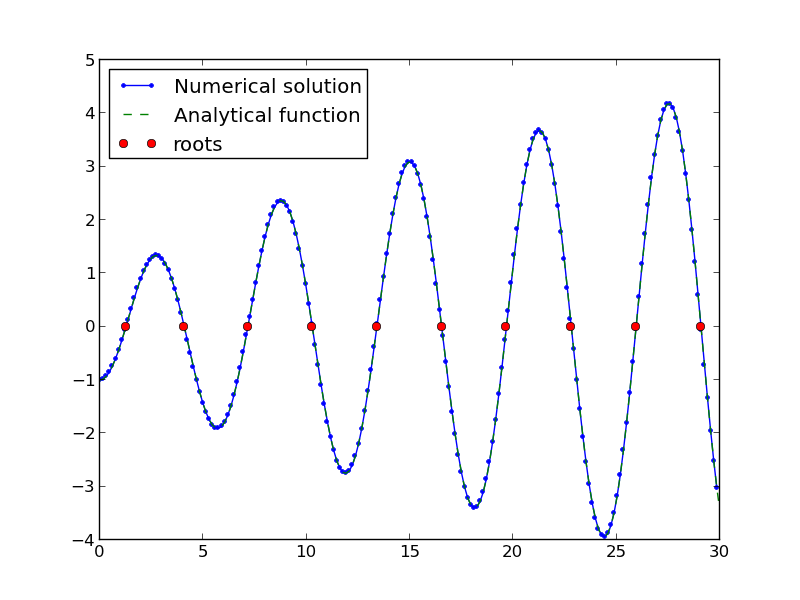## Finding the nth root of a periodic function

| categories: nonlinear algebra | tags: heat transfer

There is a heat transfer problem where one needs to find the n^th root of the following equation: $$x J_1(x) - Bi J_0(x)=0$$ where $$J_0$$ and $$J_1$$ are the Bessel functions of zero and first order, and $$Bi$$ is the Biot number. We examine an approach to finding these roots.

First, we plot the function.

from scipy.special import jn, jn_zeros
import matplotlib.pyplot as plt
import numpy as np

Bi = 1

def f(x):
return x * jn(1, x) - Bi * jn(0, x)

X = np.linspace(0, 30, 200)
plt.plot(X, f(X))
plt.savefig('images/heat-transfer-roots-1.png')You can see there are many roots to this equation, and we want to be sure we get the n^{th} root. This function is pretty well behaved, so if you make a good guess about the solution you will get an answer, but if you make a bad guess, you may get the wrong root. We examine next a way to do it without guessing the solution. What we want is the solution to $$f(x) = 0$$, but we want all the solutions in a given interval. We derive a new equation, $$f'(x) = 0$$, with initial condition $$f(0) = f0$$, and integrate the ODE with an event function that identifies all zeros of $$f$$ for us. The derivative of our function is $$df/dx = d/dx(x J_1(x)) - Bi J'_0(x)$$. It is known (http://www.markrobrien.com/besselfunct.pdf) that $$d/dx(x J_1(x)) = x J_0(x)$$, and $$J'_0(x) = -J_1(x)$$. All we have to do now is set up the problem and run it.

from pycse import *  # contains the ode integrator with events

from scipy.special import jn, jn_zeros
import matplotlib.pyplot as plt
import numpy as np

Bi = 1

def f(x):
"function we want roots for"
return x * jn(1, x) - Bi * jn(0, x)

def fprime(f, x):
"df/dx"
return x * jn(0, x) - Bi * (-jn(1, x))

def e1(f, x):
"event function to find zeros of f"
isterminal = False
value = f
direction = 0
return value, isterminal, direction

f0 = f(0)
xspan = np.linspace(0, 30, 200)

x, fsol, XE, FE, IE = odelay(fprime, f0, xspan, events=[e1])

plt.plot(x, fsol, '.-', label='Numerical solution')
plt.plot(xspan, f(xspan), '--', label='Analytical function')
plt.plot(XE, FE, 'ro', label='roots')
plt.legend(loc='best')
plt.savefig('images/heat-transfer-roots-2.png')

for i, root in enumerate(XE):
print 'root {0} is at {1}'.format(i, root)

plt.show()

root 0 is at 1.25578377377
root 1 is at 4.07947743741
root 2 is at 7.15579904465
root 3 is at 10.2709851256
root 4 is at 13.3983973869
root 5 is at 16.5311587137
root 6 is at 19.6667276775
root 7 is at 22.8039503455
root 8 is at 25.9422288192
root 9 is at 29.081221492You can work this out once, and then you have all the roots in the interval and you can select the one you want.